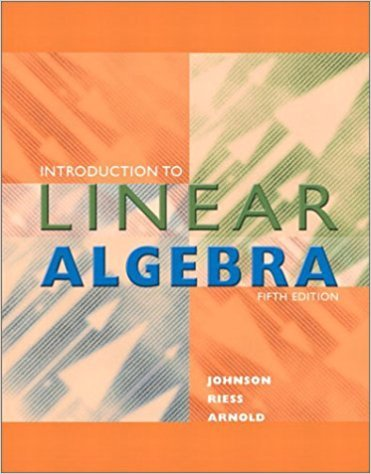×

×

Textbooks / Math / Introduction to Linear Algebra 5

# Introduction to Linear Algebra 5th Edition - Solutions by Chapter## Full solutions for Introduction to Linear Algebra | 5th Edition

ISBN: 9780201658590Introduction to Linear Algebra | 5th Edition - Solutions by Chapter

Solutions by Chapter
4 5 0 416 Reviews
##### ISBN: 9780201658590

The full step-by-step solution to problem in Introduction to Linear Algebra were answered by , our top Math solution expert on 08/03/17, 07:35AM. This expansive textbook survival guide covers the following chapters: 26. Since problems from 26 chapters in Introduction to Linear Algebra have been answered, more than 13434 students have viewed full step-by-step answer. This textbook survival guide was created for the textbook: Introduction to Linear Algebra , edition: 5. Introduction to Linear Algebra was written by and is associated to the ISBN: 9780201658590.

Key Math Terms and definitions covered in this textbook
• Back substitution.

Upper triangular systems are solved in reverse order Xn to Xl.

• Diagonalizable matrix A.

Must have n independent eigenvectors (in the columns of S; automatic with n different eigenvalues). Then S-I AS = A = eigenvalue matrix.

• Elimination matrix = Elementary matrix Eij.

The identity matrix with an extra -eij in the i, j entry (i #- j). Then Eij A subtracts eij times row j of A from row i.

• Four Fundamental Subspaces C (A), N (A), C (AT), N (AT).

Use AT for complex A.

• Left nullspace N (AT).

Nullspace of AT = "left nullspace" of A because y T A = OT.

• Length II x II.

Square root of x T x (Pythagoras in n dimensions).

• Multiplication Ax

= Xl (column 1) + ... + xn(column n) = combination of columns.

• Multiplicities AM and G M.

The algebraic multiplicity A M of A is the number of times A appears as a root of det(A - AI) = O. The geometric multiplicity GM is the number of independent eigenvectors for A (= dimension of the eigenspace).

• Multiplier eij.

The pivot row j is multiplied by eij and subtracted from row i to eliminate the i, j entry: eij = (entry to eliminate) / (jth pivot).

• Normal equation AT Ax = ATb.

Gives the least squares solution to Ax = b if A has full rank n (independent columns). The equation says that (columns of A)·(b - Ax) = o.

• Permutation matrix P.

There are n! orders of 1, ... , n. The n! P 's have the rows of I in those orders. P A puts the rows of A in the same order. P is even or odd (det P = 1 or -1) based on the number of row exchanges to reach I.

• Rank one matrix A = uvT f=. O.

Column and row spaces = lines cu and cv.

• Rotation matrix

R = [~ CS ] rotates the plane by () and R- 1 = RT rotates back by -(). Eigenvalues are eiO and e-iO , eigenvectors are (1, ±i). c, s = cos (), sin ().

• Saddle point of I(x}, ... ,xn ).

A point where the first derivatives of I are zero and the second derivative matrix (a2 II aXi ax j = Hessian matrix) is indefinite.

• Singular Value Decomposition

(SVD) A = U:E VT = (orthogonal) ( diag)( orthogonal) First r columns of U and V are orthonormal bases of C (A) and C (AT), AVi = O'iUi with singular value O'i > O. Last columns are orthonormal bases of nullspaces.

• Symmetric factorizations A = LDLT and A = QAQT.

Signs in A = signs in D.

• Triangle inequality II u + v II < II u II + II v II.

For matrix norms II A + B II < II A II + II B II·

• Vector space V.

Set of vectors such that all combinations cv + d w remain within V. Eight required rules are given in Section 3.1 for scalars c, d and vectors v, w.

• Volume of box.

The rows (or the columns) of A generate a box with volume I det(A) I.

• Wavelets Wjk(t).

Stretch and shift the time axis to create Wjk(t) = woo(2j t - k).